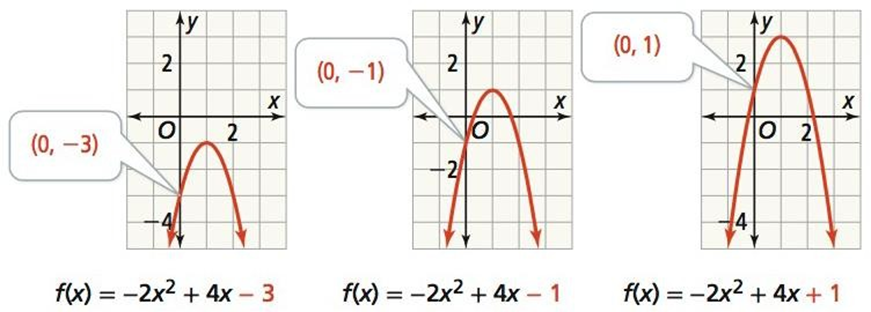Maths-
General
Easy

Question

# What information does c provide above the graph of f(x) = ax2 + bx + c## The correct answer is: f(x)=ax2+bx+c.

### Solution:- We have given 3 graphs of different functionsf(x) = -2x2 + 4x – 3f(x) = -2x2 + 4x – 1f(x) = -2x2 + 4x + 1On comparing with standard form of function f(x)=ax2+bx+c.We will get value of c as -3 , -1 , and 1 respectively in the given functions.Which gives the value of y- coordinate of point at which curve intersects y-axisWhich we call y intercept of the curve.#### With Turito Foundation.#### Get an Expert Advice From Turito.